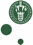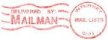McXtrace: Source_flat

The Source_flat Component

A flat rectangular or circular surface emitting x-rays

Identification

• Site:
• Author: Erik Knudsen
• Origin: Risoe
• Date: September 25, 2009

Description

A circular or rectangular xray source. Spectrum may be either gaussian or uniform around a central wavelength/energy
or read from a datafile. Xrays are considered emitted uniformly into 4pi, but a square target retricts the beam to
that window and scales the beam intensity accordingly.
If an input spectrum datafile (spectrum_file) is not specified, the beam is restricted to emit photons between E0+-dE keV, or lambda0+-dlambda AA, whichever is given.
The input spectrum file should be formatted such that x-ray energy/wavelength is in the first column and the intensity in the second. Any preceding
lines starting with # are considered part of the file header. If a datafile is given, a nonzero E0 value indicates that is is parametrized by energy (in keV)
as opposed to wavelength (in AA). Wavelength is the default.
Flux is set in the unit photons/s

Input parameters

Parameters in boldface are required; the others are optional.
 Name Unit Description Default spectrum_file File from which to read the spectral intensity profile NULL radius m Radius of circle in (x,y,0) plane where x-rays are generated. 0 yheight m Height of rectangle in (x,y,0) plane where x-rays are generated. 0 xwidth m Width of rectangle in (x,y,0) plane where x-rays are generated. Overrides xmin and xmax. 0 xmin m Lower bound of x-interval where photons are generated. 0 xmax m upper bound of x-interval where photons are generated. 0 dist m Distance to target along z axis. 1 focus_xw m Width of target .045 focus_yh m Height of target .12 focus_r 0 E0 keV Mean energy of xrays. 0 dE keV Energy half spread of x-rays (flat or gaussian sigma). 0 lambda0 AA Mean wavelength of x-rays. 0 dlambda AA Wavelength half spread of x-rays. 0 flux pht/s total flux radiated from the source 0 gauss 1 Gaussian (1) or Flat (0) energy/wavelength distribution 0 randomphase If nonzero, the phase of the emotted photon is random, i.e. source is fully incoherent. otherwise the value of phase is used. 1 phase Set phase to something given. 0

Output parameters

Parameters in boldface are required; the others are optional.
 Name Unit Description Default prms square pmul srcArea